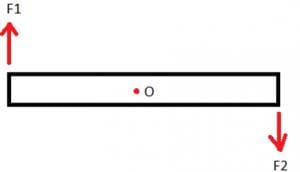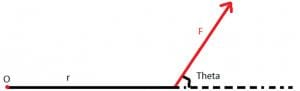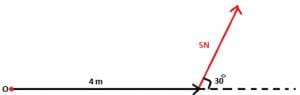Torque - Class 11

# Torque - Class 11 Notes - Class 11

## Document Description: Torque - Class 11 for Class 11 2022 is part of Class 11 preparation. The notes and questions for Torque - Class 11 have been prepared according to the Class 11 exam syllabus. Information about Torque - Class 11 covers topics like and Torque - Class 11 Example, for Class 11 2022 Exam. Find important definitions, questions, notes, meanings, examples, exercises and tests below for Torque - Class 11.

Introduction of Torque - Class 11 in English is available as part of our Class 11 preparation & Torque - Class 11 in Hindi for Class 11 courses. Download more important topics, notes, lectures and mock test series for Class 11 Exam by signing up for free. Class 11: Torque - Class 11 Notes - Class 11

Ref: https://edurev.in/question/804023/Needed-a-Document-for-torque-Related-Rotational-Mo

 1 Crore+ students have signed up on EduRev. Have you?

## What is Torque?

We can define torque as the measure of how much a force acting on an object causes that object to rotate. It is the twisting force that tends to cause rotation. The point where the object rotates is called as the axis of rotation. In physics, torque is simply the tendency of a force to turn or twist.

To explain torque in details let us consider the figure given below.We can see that the net force on the body is zero. Hence the body is in translational equilibrium. But the rod tends to rotate, this turning effect produced by force is known as moment of force or torque. Now we will consider the example of a door and try to formulate the equation for torque.

If we apply force closer to the hinge, then larger force is required to rotate the door. Also, it depends on the direction in which the force is being applied. If it is perpendicular to the line joining the hinge and the point of application of force then smaller force is required.

### Torque Formula

From the above observation, we conclude that torque produced depends on the magnitude of the force and the perpendicular distance between the point about which torque is calculated and the point of application of force. So mathematically torque is represented as:

τ = F.r. sinθUnit of torque is Newton–meter (N-m). The above equation can be represented as the vector product of force and position vector.

τ = r x F

So as it is a vector product hence torque also must be a vector. Using vector product notations we can find the direction of torque. We will consider an example to see how to calculate torque.

### Examples Of Torque

Let us consider the situation given below:In the above diagram:

= 5N

r = 4m

sinθ = 30°

Putting these values we have,

τ = 5 x 4 x sin 30°

τ = 10 N-m

The document Torque - Class 11 Notes - Class 11 is a part of Class 11 category.
All you need of Class 11 at this link: Class 11
 Use Code STAYHOME200 and get INR 200 additional OFF

Track your progress, build streaks, highlight & save important lessons and more!

,

,

,

,

,

,

,

,

,

,

,

,

,

,

,

,

,

,

,

,

,

;